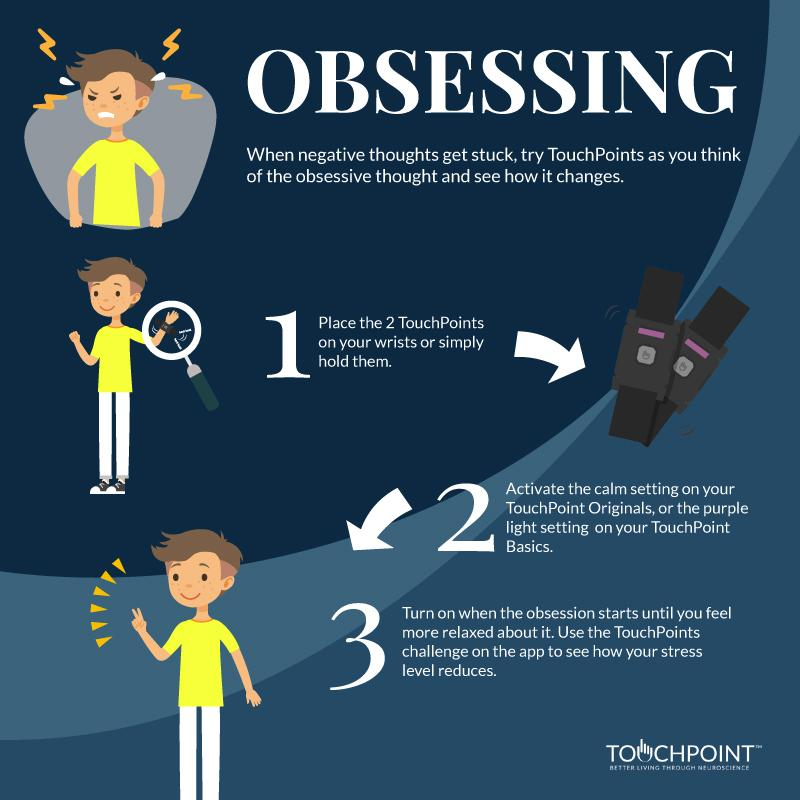0When negative thoughts get stuck, try TouchPoints as you think of the obsessive thought and see how it changes.

### When to use:

Use when the obsession starts until you feel more relaxed about it. Do the TouchPoint Challenge to see how your stress level reduces.

### Which setting to use:

 TouchPoints Original TouchPoints Basic Calm Purple

### Where to wear TouchPoints:

 Wear on wrists Hold in hands Put in pockets Slide in socks x x

<", c, ' onload="var d=', n, ";d.getElementsByTagName('head').", d, "(d.", g, "('script')).", i, "='", a.l, "'\">"].join("") } var c = "body", e = h[c]; if (!e) return setTimeout(q, 100); a.P(1); var d = "appendChild", g = "createElement", i = "src", k = h[g]("div"), l = k[d](h[g]("div")), f = h[g]("iframe"), n = "document", p; k.style.display = "none"; e.insertBefore(k, e.firstChild).id = o + "-" + j; f.frameBorder = "0"; f.id = o + "-frame-" + j; /MSIE[ ]+6/.test(navigator.userAgent) && (f[i] = "javascript:false"); f.allowTransparency = "true"; l[d](f); try { f.contentWindow[n].open() } catch (s) { a.domain = h.domain, p = "javascript:var d=" + n + ".open();d.domain='" + h.domain + "';", f[i] = p + "void(0);" } try { var r = f.contentWindow[n]; r.write(b()); r.close() } catch (t) { f[i] = p + 'd.write("' + b().replace(/"/g, String.fromCharCode(92) + '"') + '");d.close();' } a.P(2) }; a.l && q() })() }(); c[b].lv = "1"; return c[b] } var o = "lightboxjs", k = window[o] = g(o); k.require = g; k.modules = c }({}); /*]]>*/